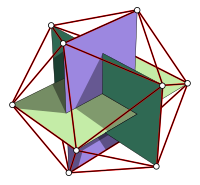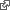#Cartesian Icosahedron

The following Cartesian coordinates define the vertices of an icosahedron with edge-length 2, centered at the origin:Icosahedron

(0, ±1, ±𝝋) (±1, ±𝝋, 0) (±𝝋, 0, ±1) where 𝝋 = (1 + √5) / 2 is the golden ratio.

Note that these vertices form three mutually centered, mutually orthogonal golden rectangles. wikiaWe offer this javascript program to enumerate the vertices.

let p = (1 + Math.sqrt(5)) / 2 rect((a,b) => [0, a*1, b*p]) rect((a,b) => [a*1, b*p, 0]) rect((a,b) => [a*p, 0, b*1]) function rect(f) { console.log(f(+1,+1)) console.log(f(-1,+1)) console.log(f(+1,-1)) console.log(f(-1,-1)) }

[ 0, 1, 1.618033988749895 ] [ 0, -1, 1.618033988749895 ] [ 0, 1, -1.618033988749895 ] [ 0, -1, -1.618033988749895 ] [ 1, 1.618033988749895, 0 ] [ -1, 1.618033988749895, 0 ] [ 1, -1.618033988749895, 0 ] [ -1, -1.618033988749895, 0 ] [ 1.618033988749895, 0, 1 ] [ -1.618033988749895, 0, 1 ] [ 1.618033988749895, 0, -1 ] [ -1.618033988749895, 0, -1 ]

We save these coordinates in a vector v. We enumerate all pairs and tally the distance² between each, confirming edge length = 2 for nearest neighbors.

6 14.47213595499958 30 10.47213595499958 30 4

We select these and print their indices.

for (let i=0; i<12; i++) for (let j=0; j<i; j++) if (edge(i,j)) console.log([i,j]) function edge(i,j) { let sq = x => x*x let d = sq(v[i]-v[j]) + sq(v[i]-v[j]) + sq(v[i]-v[j]) return d == 4 }

[ 1, 0 ] [ 3, 2 ] [ 4, 0 ] [ 4, 2 ] [ 5, 0 ] [ 5, 2 ] [ 5, 4 ] [ 6, 1 ] [ 6, 3 ] [ 7, 1 ] [ 7, 3 ] [ 7, 6 ] [ 8, 0 ] [ 8, 1 ] [ 8, 4 ] [ 8, 6 ] [ 9, 0 ] [ 9, 1 ] [ 9, 5 ] [ 9, 7 ] [ 10, 2 ] [ 10, 3 ] [ 10, 4 ] [ 10, 6 ] [ 10, 8 ] [ 11, 2 ] [ 11, 3 ] [ 11, 5 ] [ 11, 7 ] [ 11, 9 ]

Positive and Negative Feedback provides rendering ideas. We choose the tetrahedron and replace its four vertices with the icosahedron. githubhttp://ward.dojo.fed.wiki/assets/pages/cartesian-icosahedron/crazy.html HEIGHT 400

Drag to rotate.